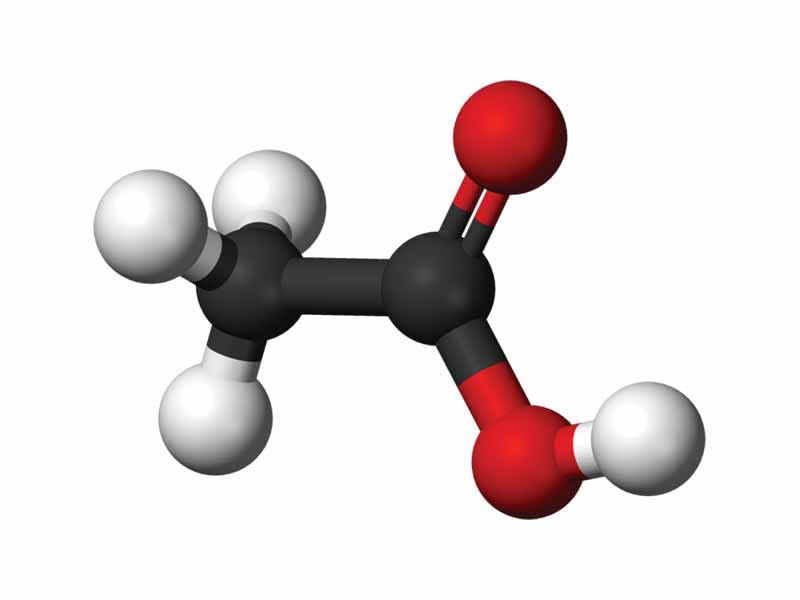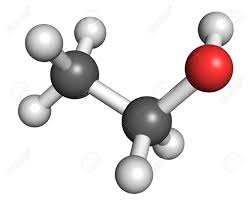CHEMISTRY STUDY GUIDE CH2 - MATTER

Complete this in a separate paper. Questions are not required. Handwritten answers only

1. What is the definition of matter?
2. Science is how you study something, not what you study. The method used is known as the scientific method. Which are the steps to the scientific method?
3. What is the purpose of having a control or control group?
4. What is the minimum number of times an experiment should be done?
5. What is a hypothesis?
6. A variable?
7. A placebo?
8. What is the difference between qualitative and quantitative data?
9. Matter is classified based on its physical and chemical properties
1. What are chemical properties?
2. What are physical properties?
10. A compound is a pure substance that consists of chemically bonded atoms of two or more____
11. Classify the following changes as physical or chemical.
1. Silver tarnishes when it combines with sulfur to form silver sulfide, which is black.
2. When ignited, phosphorus burns in air to form P4O10, a compound of phosphorus and oxygen.
3. Rubbing alcohol evaporates from your skin, and the alcohol vapor mixes with the air.
4. Limestone, calcium CaCO3, gives off carbon dioxide when heated and changes to a different compound, calcium oxide.
1. In terms of particles, the major difference between a liquid and a gas is_______________
2. In terms of particles, the major difference between a solid and a liquid is______________
3. What are the characteristic properties of solids?
4. What are the characteristic properties of liquids?
5. What are the characteristic properties of gases?
6. Why solids and liquids cannot be compressed, but gases can?
7. Classify the following properties of iron as chemical or physical
1. One cubic centimeter of iron has a mass of 7.86 g.
2. Iron melts at 1535°C.
3. Iron can be hammered into different shapes.
4. Iron reacts with hydrochloric acid to produce hydrogen gas and iron(III) chloride.
1. Classify the following as pure elements or mixtures?
1.  sawdust
2. brass
3. sea water
4. gold
5. table sugar (sucrose)
6. aluminum
7. water
8. salt solution
1. All samples of aluminum sulfate, a compound, have the same characteristic properties and the same ________________.
2. What is the main characteristic of a mixture?
3. Define “solution” and give three examples.
4. Give the name of the main fractions in the crude oil fractional distillation process.
5. A precipitate formed in the following experiment done in the petri dish below. What has to happen for the precipitate to form?

6.  write the molecular formula for the following stick and ball representations
The black represents Carbon (C), the red represents Oxygen (O) and the white represents Hydrogen (H)

a)b)c)d)7.  Describe diffusion in gases.
8.  You need to calculate the density of an irregular solid. List the equipment you would need to do that.
9.  Classify the following pictures as Pure substances (Include if they are elements or compounds) or mixtures10.  Define exothermic and endothermic reactions
11.   A metal melts at 800 °C and boils at 2500 °C sketch the heating curve for the metal if you begin heating it at room temp (20 °C) and you end at 2800°C.
12.  On the graph you sketched, mark the states of the matter present in each segment
13.  On the graph you sketched, mark the melting and boiling process.
14. How is the heat used in the flat sections of the curve?
15.  which method would you use to separate:
1. water from salt (retrieving both substances)
16.
17.
18.
19.
20.
21.